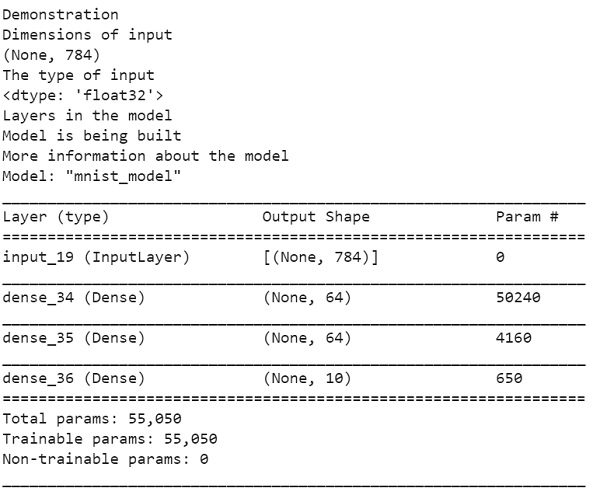# Discuss how the Keras functional API can be used to create layers using Python

Tensorflow is a machine learning framework that is provided by Google. It is an open-source framework used in conjunction with Python to implement algorithms, deep learning applications and much more. It is used in research and for production purposes. It has optimization techniques that help in performing complicated mathematical operations quickly.

The ‘tensorflow’ package can be installed on Windows using the below line of code −

pip install tensorflow

Keras means ‘horn’ in Greek. Keras was developed as a part of the research for the project ONEIROS (Open-ended Neuro-Electronic Intelligent Robot Operating System). Keras is a deep learning API, which is written in Python. It is a high-level API that has a productive interface that helps solve machine learning problems. It runs on top of the Tensorflow framework. It was built to help experiment in a quick manner. It provides essential abstractions and building blocks that are essential in developing and encapsulating machine learning solutions.

Keras is already present within the Tensorflow package. It can be accessed using the below line of code.

import tensorflow
from tensorflow import keras

The Keras functional API helps create models that are more flexible in comparison to models created using sequential API. The functional API can work with models that have non-linear topology, can share layers and work with multiple inputs and outputs. A deep learning model is usually a directed acyclic graph (DAG) that contains multiple layers. The functional API helps build the graph of layers.

We are using the Google Colaboratory to run the below code. Google Colab or Colaboratory helps run Python code over the browser and requires zero configuration and free access to GPUs (Graphical Processing Units). Colaboratory has been built on top of Jupyter Notebook. Following is the code snippet wherein we will see how the Keras functional API can be used to create layers using Python −

## Example

import numpy as np
import tensorflow as tf
from tensorflow import keras
from tensorflow.keras import layers

inputs = keras.Input(shape=(784,))
print("Demonstration")
img_inputs = keras.Input(shape=(32, 32, 3))
print("Dimensions of input")
print(inputs.shape)
print("The type of input")
print(inputs.dtype)
print("Layers in the model")
dense = layers.Dense(64, activation="relu")
x = dense(inputs)
x = layers.Dense(64, activation="relu")(x)
outputs = layers.Dense(10)(x)
print("Model is being built")
model = keras.Model(inputs=inputs, outputs=outputs, name="mnist_model")
model.summary()

Code credit − https://www.tensorflow.org/guide/keras/functional

## Output## Explanation

• An input node is created, and the shape of the data is set to a 784-Dimensional vector.

• The input that is returned contains information about the ‘shape’ and ‘dtype’ of input data which was previously fed to the model.

• A model is created by specifying the inputs and outputs.